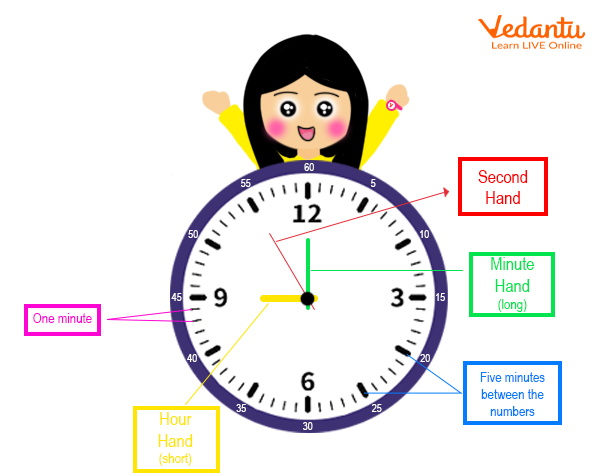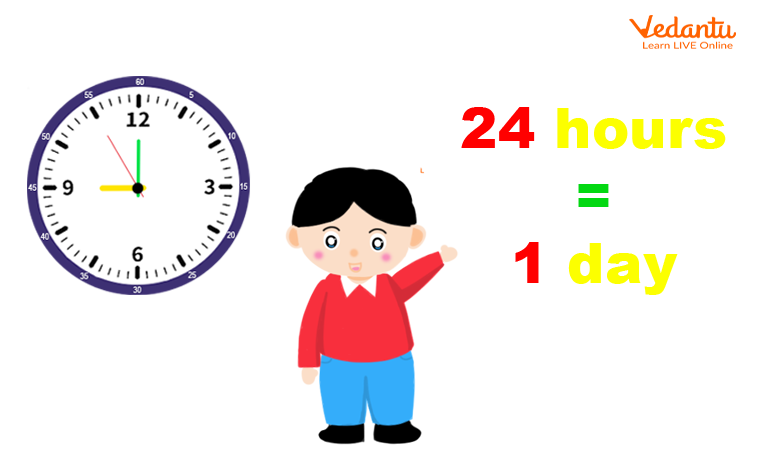Courses
Courses for Kids
Free study material
Free LIVE classes
More

# How to Convert Hours into SecondsLIVE
Join Vedantu’s FREE Mastercalss

## About: Hours and Seconds conversion

Searching for a comprehensive tutorial to help you convert hours to seconds? then you've come to the right page. Seconds and hours are both used as units to measure time. With cases that have been resolved, we have fully detailed the hours-to-seconds conversion here. You can also look at the math conversion chart on our website to have a better understanding of how to convert length, mass, and capacity along with time. Check out the lessons below to learn the definitions of an hour and a second, a straightforward conversion formula, and worked examples of converting an hour to a second.A Clock

### What is an Hour?

Depending on the speed of the Earth's rotation, an hour is a measure of time that is traditionally calculated as $\dfrac{1}{{24}}$ of a day. Have you ever thought how many seconds are there in 1 hour? 1 hour in seconds is scientifically estimated to be 3600 seconds. A day consists of 24 hours and there are 60 minutes in an hour. An hour is sometimes also represented as “hrs”.

### What is Second?

The International System of Units (SI) defines the second (symbol: s) as 186400 of a day. This component was determined from the division of a day into 24 hours, 60 minutes, and then 60 seconds.

### Calculate 1 hour in Seconds

1 Hour $= 60$ Minute

1 Minute $= 60$ Seconds

Therefore,

1 Hour $= 60 \times 60$ Seconds

$= 3600$ seconds

On inversing the relation we will get the relationship between seconds and hours.

### How many Seconds are there in One 1 Hour?

There are 3600 seconds in 1 hour.

Mathematically, it can be shown as follows:

1 hour  $= 3600$ seconds

### How to Convert Hours into Seconds Example:

Convert 6 hours into seconds

As we know,

1 hour $= 3600$ seconds

Thus,

6 hours $= 6 \times 3600$ seconds

$= 21600$ seconds

Therefore 6 hours $= 21600$ seconds

• The Minute hand is the longest needle in the watch.

• The Hour Hand is the shortest needle in the watch.

• The Fastest moving needle is the second hand.

• There is a 5 minute difference in all the numbers from 1 to 12.• 24 hours is equal to one day.24 Hours=1 day.

### Conclusion:

The series of ongoing occurrences that make up time. including the past, present, and future. The second is the basic unit of time. Additional terms that we consider include hours, minutes, days, weeks, months, and years. Clocks can be used to measure time. We have also learned interesting facts about the era from our studies.

### Solved Examples:

1: Convert 42 hours into minutes?

Ans: As we know,

1 Hour = 3600 Seconds

Hence, 42 Hours = 4 X 3600

= 14400 Seconds.

2: Calculate the seconds in 45 mins?

Ans: As we know,

1 minute = 60 seconds

Hence, 45 Minutes = 45 X 60

= 2700 Seconds.

3. Convert 45 hours and 56 minutes into seconds.

Ans: As we know,

1 Hour = 60 Minutes

Hence, 45 hours = 45 X 60

= 2700 Minutes.

Total minutes=2700 + 56

= 2756 minutes.

And 1 Minutes = 60 Seconds

Hence, 2756 minutes = 2756 X 60

= 165360 Seconds.

Therefore, 45 hours and 56 minutes are equal to 165360 seconds.

Last updated date: 28th Sep 2023
Total views: 64.2k
Views today: 2.64k

## FAQs on How to Convert Hours into Seconds

1. Why Do 24 hours equal One day?

Accordingly, the sun takes 24 hours to take one round of sun, including day and night. That’s the reason they are there 24 hours a Day.

2. How many seconds are there in a lifetime?

A lifespan consists of about 22,075,000 seconds.

3. How many hours are there in 3 days?

There are 72 hours in 3 days.

As there are 24 hours in one day. In 3 days there are $24 \times 3$ hours.

4. How many hours are there in a week?

There are 168 hours in a week.